## Volume 13 - Issue 2

Research Article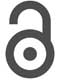Creative Commons, CC-BY

# Dissociation Energy of an (O2) Oxygen Molecule by DFT Calculations

*Corresponding author: Juan Horacio Pacheco Sánchez, TecNM / Technological Institute of Toluca / Division of Graduate Studies and Research, Metepec, 52149, Edo. Mex., Mexico.

Received: May 24, 2021; Published: June 14, 2021

#### Abstract

Dissociation energy of an oxygen molecule is an intense research using platinum as catalyst against not only carbon electrodes of fuel cells but also hypoxia which is low oxygenation in blood. Our aim is to exhibit platinum at least in four different complexes interacting with oxygen molecule through DFT calculations in designing intermediate molecule complexes for treatment of this kind of applications. Reaction mechanisms among oxygen molecules and platinum catalysts (Pt, Pt4) are also described in interaction with C60 activated carbon as electrode of a fuel cell by means of DFT calculations. This represents a bridge bond between the theoretical calculations and the processes of fuel transformation in the fuel cells (PEMFC). Moreover, these platinum complexes can be used to build new carboplatin molecules against hypoxia. Our basic results match with DFT calculations supported on experimental data. In addition, the catalytic support C60Pt4 breaks the oxygen molecule O2, consequently it is found that hrH breaks before vrH and this before drH.

Keywords: Blood Cancer, Catalytic Interaction Platinum-Oxygen, Activated Carbon through Fullerene (C60), Fuel cells

#### Introduction

The aim of this work is the breakdown of the O2 molecule from a platinum catalyst (Pt), a platinum surface (Pt4) and a catalytic support (C60Pt4) using DFT calculations. For cancer therapy in the late 1970s, cisplatin has been used so widely that it is often called ‘‘penicillin of cancer’’. The continued success of this chemotherapeutic drug inspired the further development of platinum compounds for anticancer therapy, generating hundreds of cisplatin analogs. Nevertheless, platinum complexes are promising anticancer agents, as they show high stability and bioavailability, allowing oral application and overcoming side effects such as nephrotoxicity [1,2]. Platinum complexes must be activated by reduction to release their active platinum metabolites. Therefore, platinum complexes, as bioreductive prodrugs, might target hypoxia and close the gap between classic metal-based and targeted therapies [3,4]. Moreover, platinum complexes can be used to build new carboplatin molecules against hypoxia. As an example, carboplatin-loaded chitosan nanoparticles (CRB NP) have been proposed to increase the cancer treatment efficiency in wilm’s tumor . In addition, the platinum (supported by high-surface area carbons, i.e. Pt/C) is employed as the main electrocatalyst material in both anode and cathode  where there are reaction mechanisms between the Pt catalyst and one of the fuels (O2), and for a good reason platinum is the best single element catalyst in acidic media for the hydrogen oxidation reaction (HER) and the oxygen reduction reaction (ORR) taking place on the two electrodes, respectively [7,8]. The widespread application of platinum in electrocatalysis is due to its relatively good stability [9,10] on fuel cells of PEM kind. Previously, preliminary ab initio calculations on the Pt(3D,1S)-O2 interaction have been accomplished . The reason we need for calculating over and over the Pt-O2 interaction thus studied is that we need to understand more clearly the most part of its reaction mechanisms. This is pursued with the intention to design fuel cells among other applications . DFT theoretical calculations provide useful information for designing processes of fuel transformation in the fuel cells.

#### Computational Method

In this work, DFT calculations are achieved through Biovia Materials Studio Dmol3 software [13,14] The exchange and correlation function are applied using the generalized gradient approximations (GGA), and Perdew Burke and Ernzerhof (PBE)  functional, which provides good results, with a set of double bases (dnd). The set of bases have the advantage of being equivalent to the Gaussian base 6-31G* for restricted spin . All structures were optimized until the forces were less than 0.002 Ha / Å. DMol3 module is used to calculate total energy, where energies are obtained by single point calculations with Hartree-Fock method . Connectivity calculation of BIOVIA Materials Studio DMol3 on no-bonding to s- and f-shell scheme, bond type, and converting representation to Kekulé, for bond length tolerances from 0.6 to 1.15 Å is a software-tool to bond atoms according to their separation distance, which has been applied for building possible reactivity products .

#### Results and Discussion

##### Interaction of the Platinum atom with an Oxygen atom (Pt-O)

It was considered to carry out the Pt-O interaction as input in the simulation as shown in Figure 1 where a starting distance of 3.16 Å has been proposed to start with geometry optimization calculation.

The output result of the geometry optimization produced an equilibrium distance of 2.06 Å (Figure 2) and an equilibrium energy of -13.2992 eV.

The connectivity calculation was carried out and we got the corresponding bond as shown in Figure 3.Figure 1: Molecular arrangement of the platinum atom with the oxygen atom (input).

Once the distance and equilibrium energy were obtained, one of the two atoms was fixed, in this case it was the platinum atom, the oxygen atom moved away step by step with each step of 0.1Å until reaching a constant energy at an infinite separation distance, at each position of the oxygen atom its energy is calculated, and thus we construct the curve of the potential energy well. Table 1 exhibits distances and energies obtained for the construction of the potential energy well, which is 3.745eV size as shown in Figure 4.

Comparing the energy of the potential well of the Pt-O interaction, which is 3.745eV in our simulation, and the experimental value of 3.82eV  there is an error of 1.4%.

##### Interaction of the Platinum atom with an Oxygen molecule (Pt-O2)

The geometry optimization of the oxygen molecule (O2) calculation, with an O = O bond distance of 1.21Å in the simulation, has been compared with an experimental value of 1.25Å  as shown in Figure 5.

The geometry optimization calculation of platinum atom with oxygen molecule provides the equilibrium energy, and at the input, the platinum atom is placed at a separation distance of 3.5Å from the oxygen molecule, to carry out the calculation (Figure 6).

Geometry optimization gives an equilibrium distance of 2.049Å and an equilibrium energy of -18.838 eV. The connectivity calculation was accomplished (Figure 7).

The potential energy well of the Pt-O2 interaction is calculated as shown in Figure 8. Where the platinum atom is fixed, and the oxygen molecule is moved away and closer from 0.1Å to 0.1Å. At each distance, an energy value is obtained as shown in Table 2, for which a potential well size of 0.725eV has been determined. The experimental value of this interaction is equivalent to 0.719eV . Then, the approximation error is 1% between the calculated and experimental value (Figure 8).

The PtO2 intermediate complex is taken to break an oxygen atom and free it with PtO + O products. Table 2 of the potential energy of PtO2 has an equilibrium distance of 2.049Å, an equilibrium energy -18.838eV, and a potential energy well depth 0.725eV. Previous equilibrium distance is shown in Figure 7, which also exhibits 1.32Å for O-O bond length, and it must be noticed that the well depth value agrees with the energy obtained by a fuel mono cell [20-22].

From the already optimized system we fixed two atoms, the platinum atom, and the oxygen atom, later an oxygen atom is moved away due to it is not fixed. It is moved horizontally until the energy is constant. For each distance, an energy value is obtained as shown in Table 3 (Figure 8).

The potential energy curve is constructed, and the well size is found to be 3.646eV (Figure 10). This agrees with potential energy well depth of the Pt-O interaction calculated previously (Table 3).

The already optimized Pt + O2 system was taken up again now as PtO2, then fixing two atoms, one of platinum and one of oxygen, later an oxygen atom that is not fixed is vertically moved away step by step until the energy is constant. For each distance, an energy value is obtained as shown in Table 4 (Figure 11&12).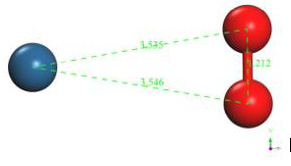Figure 6: Molecular arrangement of the platinum atom with the oxygen molecule (input).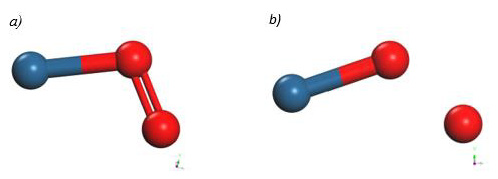Figure 9: a) Moving the oxygen atom away horizontally, b) Breaking one of the Pt-O bonds.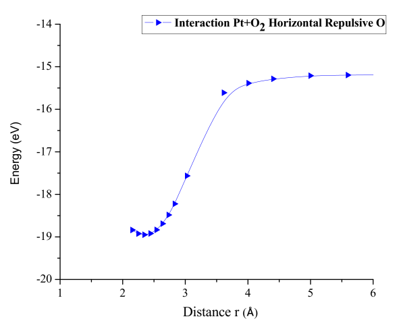Figure 10: Potential energy curve of the breaking of the Pt-O bond, moving the oxygen atom away horizontally.Figure 11: a) Moving the oxygen atom away vertically, b) Breaking one of the Pt-O bonds.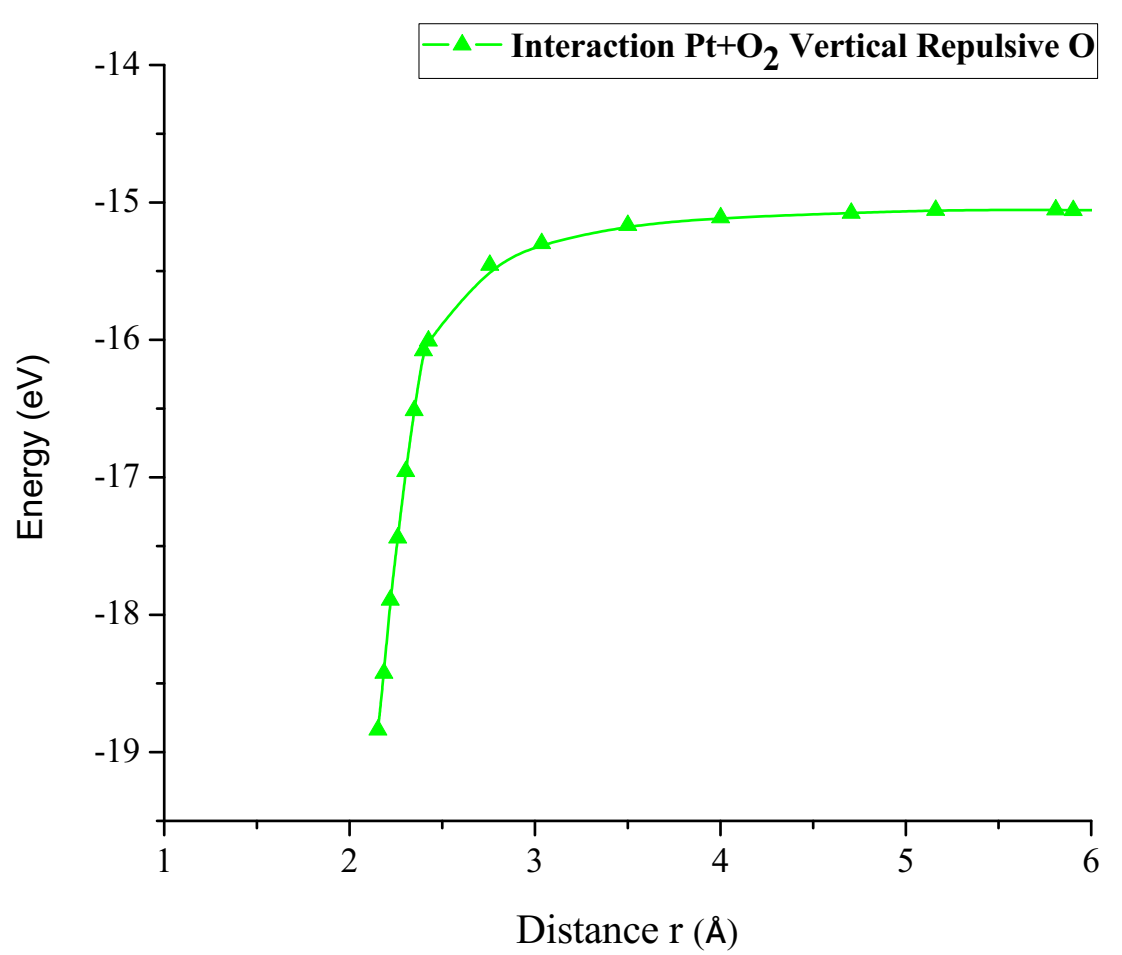Figure 12: Potential energy curve of the breaking of the Pt-O bond, moving the oxygen atom away vertically.

The energy curve for breaking the O2 molecule was constructed vertically, it was found that potential well size is 3.783eV as shown in Figure 12. This agrees with potential energy well depth of the Pt-O interaction calculated previously.

Finally, the optimized Pt + O2 system is taken again, by fixing platinum and oxygen atoms, and the other oxygen atom is moved away diagonally until the energy calculated is constant (Figure 13). For each distance, an energy value is obtained as shown in Table 5.

The energy curve for breaking the O2 molecule is now constructed diagonally, and the well size is 3.638eV (Figure 14). Which agrees with the previous potential energy well depth of Pt-O interaction calculated (Table 5).

In Figure 15 it is found that the inflection points, vertical repulsive oxygen (vrH) = 3.783Å, diagonal repulsive oxygen (drH) = 3.638Å, horizontal repulsive hydrogen (hrH) = 3.757Å, consequently it is found that drH breaks before hrH and this before vrH. And with energies vrH = 3.783eV, drH = 3.638eV, hrH = 3.757eV, these correspond to what it takes on average almost the same amount of energy to dissociate a Pt-O bond. This agrees with potential energy well depth of the Pt-O interaction calculated previously.Figure 13: a) Diagonally moving the oxygen atom away, b) Breaking of one of the Pt-O bonds.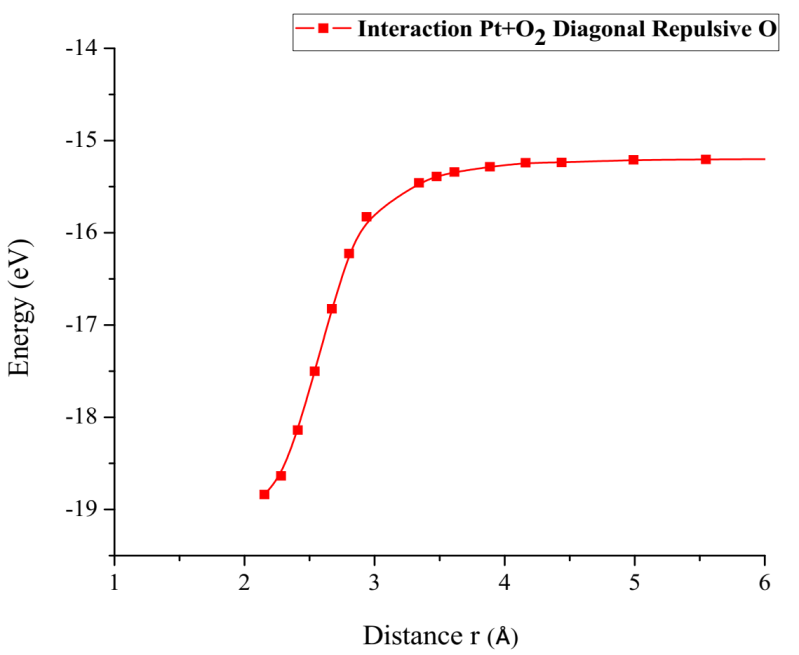Figure 14: Potential energy curve of the breaking of the Pt-O bond, moving the oxygen atom away vertically.

##### Interaction of a Platinum Cluster with an Oxygen molecule (Pt4-O2)

The output of the geometry optimization of platinum cluster (Pt4), which has a Pt-Pt bond distance of 2.712Å in the simulation as shown in Figure 16, agrees with the experimental value of 2.68Å .

The geometry optimization calculation of the platinum cluster is accomplished with the oxygen molecule. A the input, the platinum cluster is placed at a distance of 4.176Å from the oxygen molecule to carry out the optimization (Figure 17).

The geometry optimization gives an equilibrium distance of 2.295Å and an energy of -49.092 eV. The connectivity calculation is applied as shown in Figure 18.

The potential energy well of the Pt4-O2 interaction is calculated as shown in Figure 19, considering fixed the platinum cluster, and the oxygen molecule is moved away and closer from 0.1Å to 0.1Å. At each distance, an energy value is obtained as shown in Table 6, for which a potential well size of 0.646eV is obtained. The experimental value of the interaction is equivalent to 0.669eV , and there is an error of 3.5%.

The Pt4O2 complex formed at the minimum of the potential energy curve is broken by taking out one oxygen atom to free it, to obtain Pt4O + O as products. The Pt4O2 complex molecule has an equilibrium distance of 2.295Å between Pt4 and O2, and an equilibrium energy of -49.092eV with an energy well depth 0.646eV potential as shown in Figure 19. The output after geometry optimization gives 1.3Å for O-O bond length,

From the already optimized system we fixed the platinum cluster and one oxygen atom, later the oxygen atom not fixed is moved away horizontally until the energy is constant (Figure 20).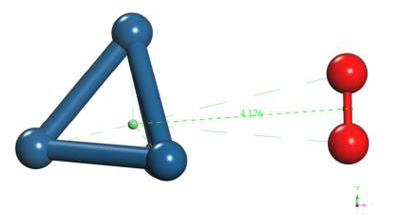Figure 17: Molecular arrangement of the platinum cluster with the oxygen molecule (input)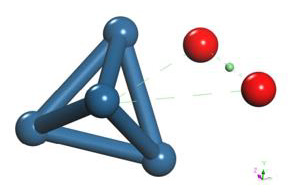Figure 20: Moving the oxygen atom away horizontally and breaking the O2 molecule.

As shown in Figure 20, the platinum cluster breaks the O2 molecule as it moves horizontally towards and away an oxygen atom. The energy curve was constructed, in each distance an energy value was obtained as shown in Table 7, it was found that the well size is 3.586eV (Figure 21).

The already optimized Pt4 + O2 system is taken again, fixing the platinum cluster and an oxygen atom, later an oxygen atom not fixed is vertically moved away until the energy is constant (Figure 22).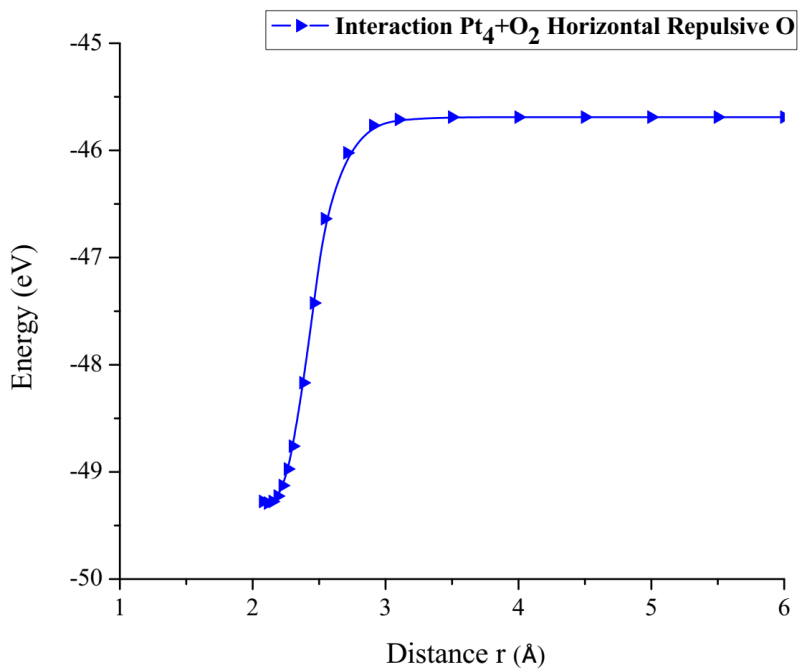Figure 21: Potential energy curve of the breakdown of the O2 molecule, moving the oxygen atom away horizontally.

The energy curve for breaking the O2 molecule was constructed vertically, at each distance an energy value is obtained as shown in Table 8, it was found that the well size is 3.668eV (Figure 23).

Finally, the optimized Pt4 + O2 system was taken up again, we fixed the platinum cluster and an oxygen atom, later the oxygen atom began to move away, which was not fixed diagonally until the energy was constant (Figure 24). At each distance, an energy value was obtained as shown in Table 9.

The energy curve for breaking the O2 molecule was constructed diagonally, and the well size was found to be 3.617eV (Figure 25).

In Figure 26 it is found that the inflection points, vertical repulsive oxygen (vrH) = 3.668Å, diagonal repulsive oxygen (drH) = 3.617Å, horizontal repulsive oxygen (hrH) = 3.586Å, consequently it is found that hrH breaks before drH and this before vrH, and with the energies vrH = 3.668eV, drH = 3.617eV, hrH = 3.586eV. These correspond to what it takes on average almost the same amount of energy to break the oxygen molecule O2.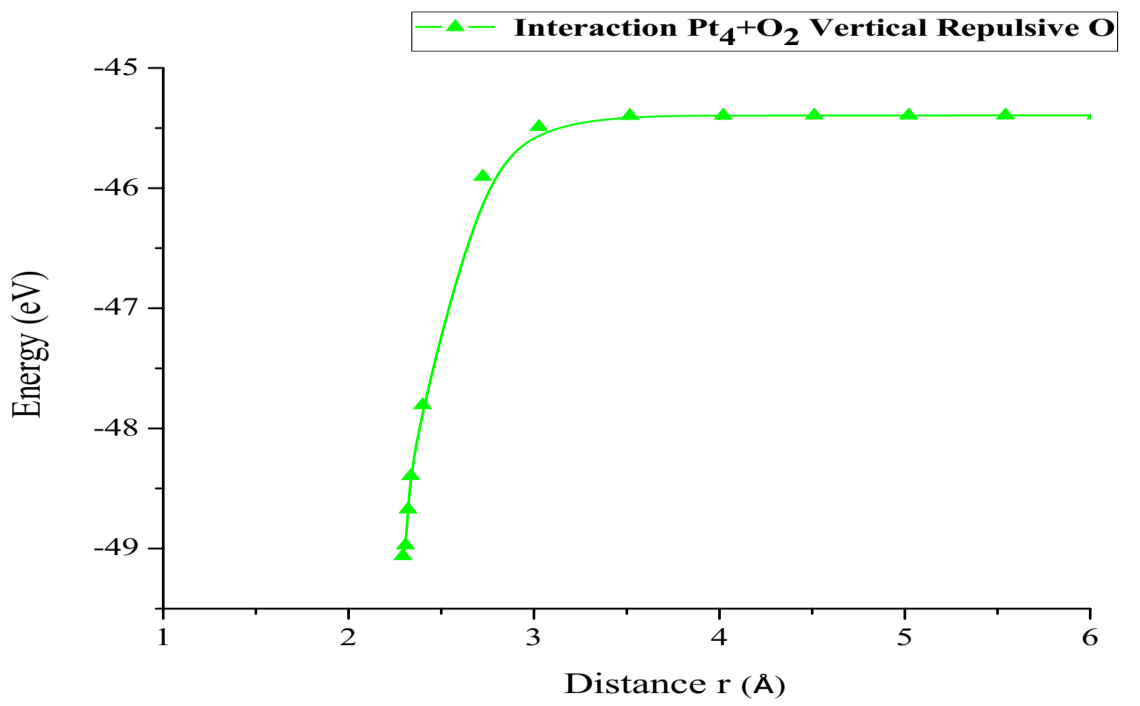Figure 23: Potential energy curve of the breakdown of the O2 molecule, moving the oxygen atom away vertically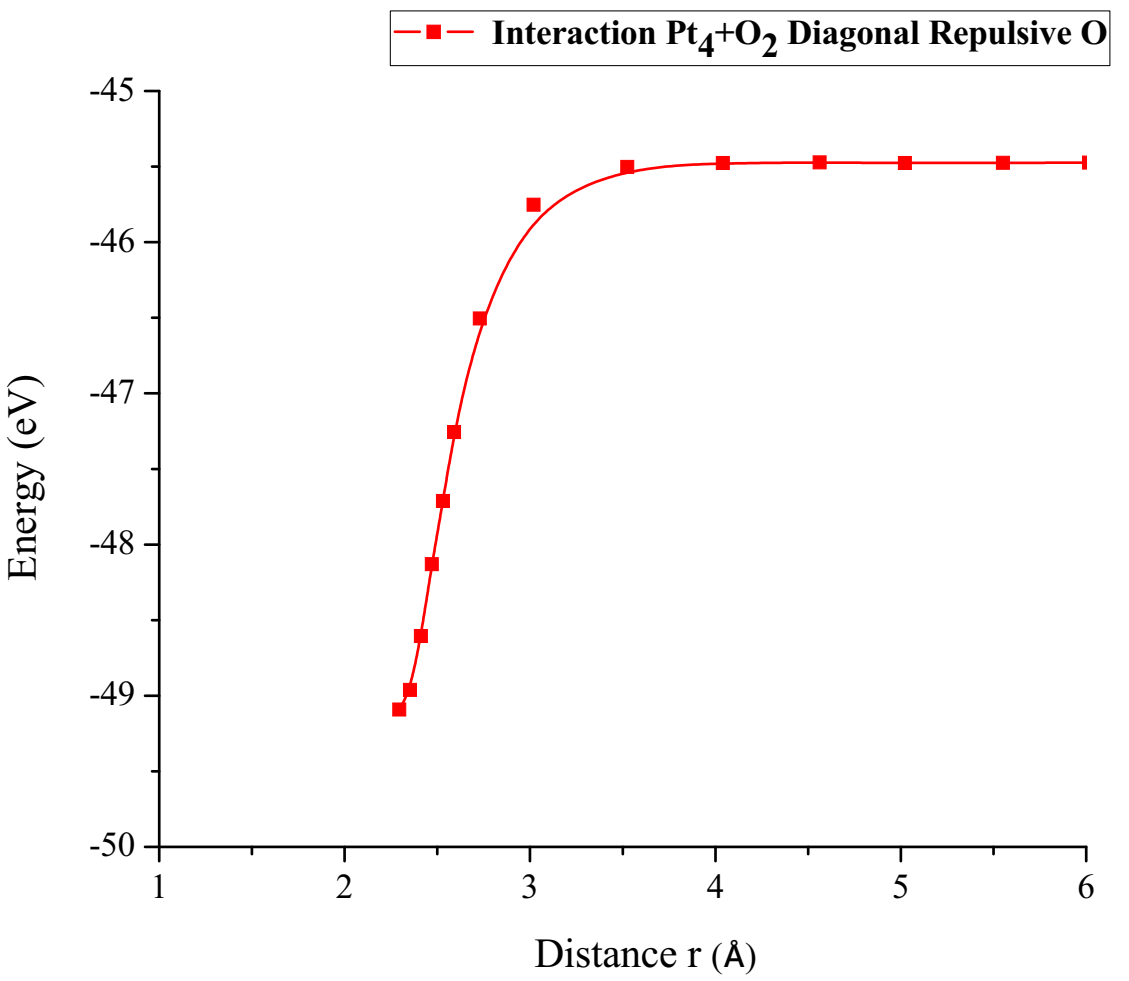Figure 25: Potential energy curve of the breakdown of the O2 molecule, moving the oxygen atom away diagonally.

##### Interaction of the C60Pt4 System with an Oxygen molecule (C60Pt4-O2)

The C60Pt4 system was placed at 1.45 Å from the O2 molecule as shown in Figure 27.

The geometry optimization calculation of the C60Pt4 system is accomplished with the oxygen molecule. The geometry optimization gives an equilibrium distance of 2.048Å and an energy of -451.2475 eV, and the connectivity calculation is applied (Figure 28).

The potential energy well of the C60Pt4-O2 interaction is calculated as shown in Figure 29, and for this the C60Pt4 system is set fixed, and the oxygen molecule is moved away and closer from 0.1Å to 0.1Å. At each distance, the corresponding energy value is obtained as shown in Table 10, for which a potential well size of 0.617eV is obtained, the experimental value of the interaction is equivalent to 0.619eV  there is an error of 0.3%, then the agreement is very good.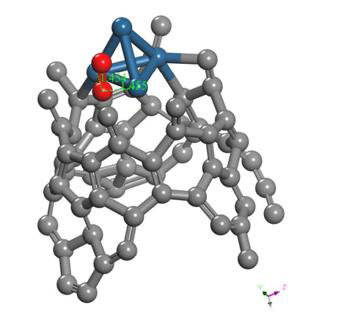Figure 27: Molecular arrangement of the C60Pt4 system with the oxygen molecule (input).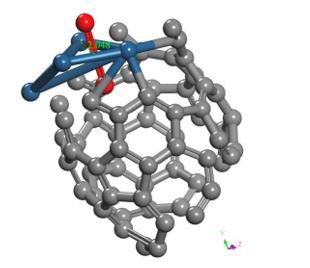Figure 28: Geometry optimization of the molecular arrangement C60Pt4-O2 (output) of an intermediate complex.

The C60Pt4-O2 system is carried out to break the oxygen molecule, for obtaining C60Pt4O + O products. Having Started with C60Pt4-O2 system already geometrically optimized (Figure 28), which has an equilibrium distance of 2.048Å between C60Pt4-O2 and an equilibrium energy -451.2475eV with a potential energy well depth of 0.617eV.

From the already optimized system we fixed the C60Pt4 system and an oxygen atom, later the non-fixed oxygen atom is moved away horizontally until the energy is constant. At each distance, the corresponding energy value is obtained, as shown in Table 11 (Figure 30).

As shown in Figure 30, the C60Pt4 system breaks the oxygen molecule (O2) when step by step one oxygen atom is horizontally moved away and closer. The energy curve is constructed and the potential well size is found to be 2.766 eV (Figure 31) (Table 11).

The already optimized C60Pt4-O2 system is taken up again, fixing the C60Pt4 system and an oxygen atom, later an oxygen atom that was not fixed vertically began to move away until the energy was constant (Figure 32).

At each distance, an energy value is obtained as shown in Table 12, which is used to vertically build the energy curve for breaking the O2 molecule. It was found that the well size is 2.798eV (Figure 33).

Finally, the optimized C60Pt4-O2 system was taken again, we fixed the C60Pt4 system and an oxygen atom, later the other oxygen atom is diagonally moved away, until the energy is constant (Figure 34).

At each distance, an energy value was obtained as shown in Table 13, the energy curve for breaking the O2 molecule is diagonally constructed, and the well size is found to be 2.829eV (Figure 35).

In Figure 36, for the inflection points, vertical repulsive oxygen (vrH) = 2.798Å, diagonal repulsive oxygen (drH) = 2.829Å, horizontal repulsive oxygen (hrH) = 2.766Å, it is found that hrH breaks before vrH and this before drH, with energies vrH = 2.798eV, drH = 2.829eV, hrH = 2.766eV, respectively. These values agree with the same amount of energy to dissociate the oxygen molecule O2.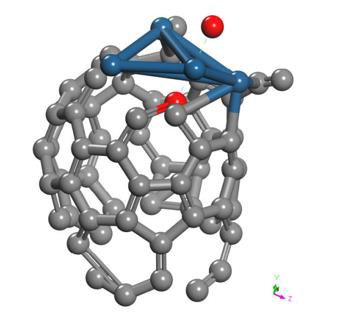Figure 30: Moving the oxygen atom away horizontally for breaking the O2 molecule.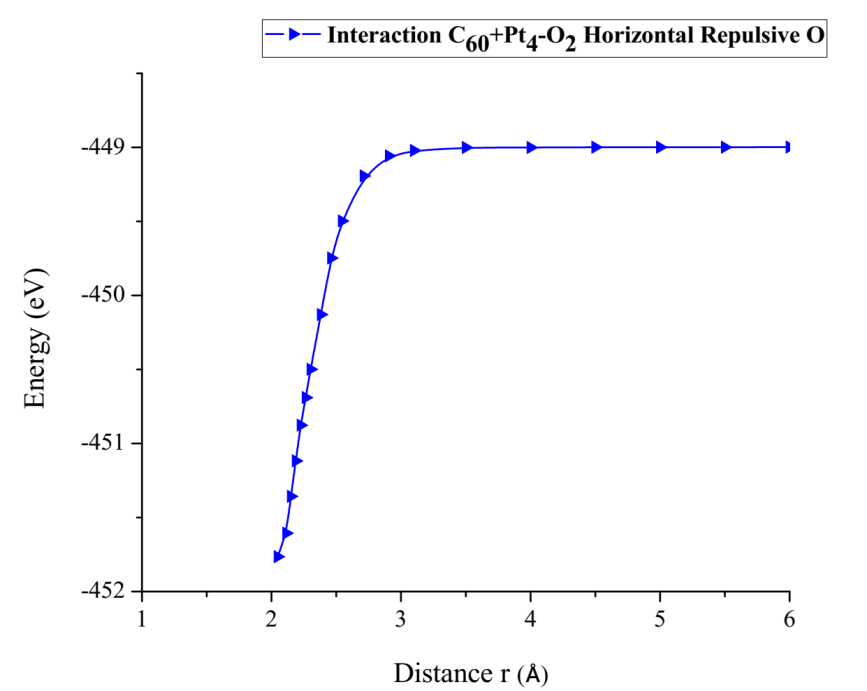Figure 31: Potential energy curve of the breakdown of the O2 molecule, moving the oxygen atom away horizontally.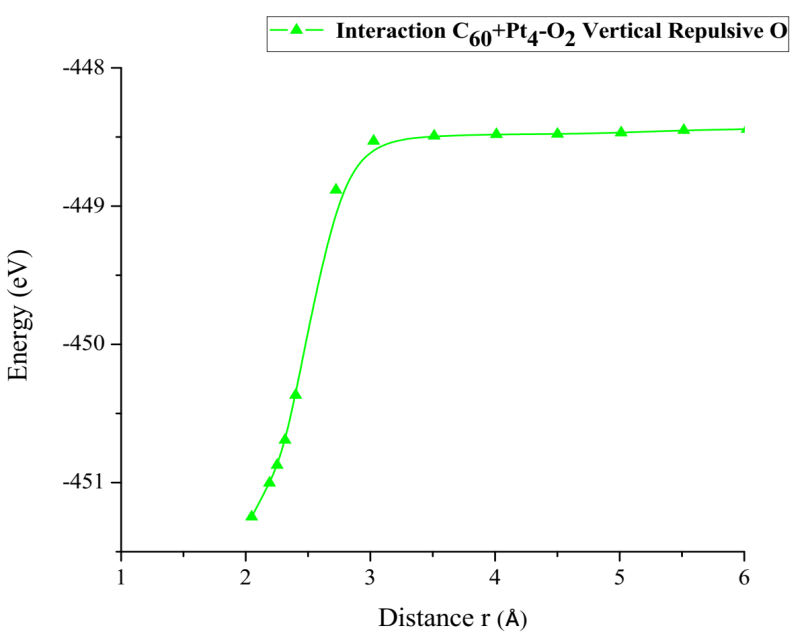Figure 33: Potential energy curve of the breakdown of the O2 molecule, moving the oxygen atom away vertically.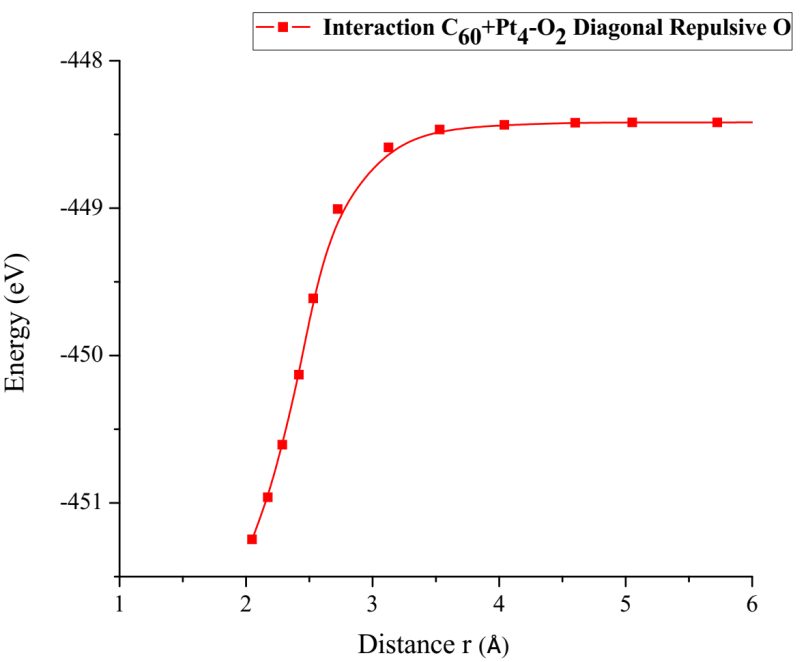Figure 35: Potential energy curve of the breakdown of the O2 molecule, moving diagonally the oxygen atom away.Figure 36: Comparison of interactions for breaking an oxygen atom in several configurations.

#### Conclusions

Our results match with DFT calculations supported on experimental data. The interaction among oxygen molecules in different configurations and different forms of platinum has application in this case on hypoxia which is a blood cancer and on electrodes of fuel cells through calculated energies. DFT calculations might help in designing intermediate molecule complexes for treatment of this kind of hypoxia cancer. In addition, the catalytic support C60Pt4 breaks the oxygen molecule O2, and it is found that hrH breaks before vrH and this before drH. With energies hrH = 2.766eV, vrH = 2.798eV, drH = 2.829eV, these correspond to what it takes on average almost the same amount of energy to dissociate the oxygen molecule O2.